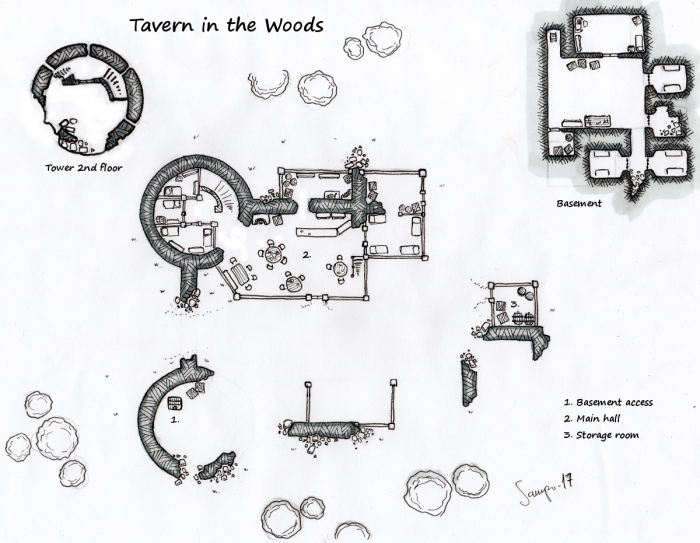## Map – Tavern in the WoodsHere’s another hand drawn map of a tavern build on ruins of an old keep. It’s located in remote woods away from the most frequented roads. Usual customers are hunters, smugglers and bandits. There is a strict policy of not asking questions and both the keeper and clientele like to keep disputes away from the tavern. Indeed, rare aggressors usually find themselves facing an unlikely alliance.

The tavern itself has a main room with almost around the clock service. The tower has a couple of rooms offering privacy but most opt for a simple shared accommodation. Besides the main hall, there is a basement. It has a couple of holding cells but most of the time it is used simply for storage or even accommodation.

The fist case is the so called exploding dice, i.e. if a die comes up with the (usually) maximum possible result, then another die is rolled and added to the dice pool. Eg. a player rolls 5 d6s, and gets 1,1,3,6,6. The player then rolls two more d6s, say 2,6. The second six again adds another die which gives 2. Given the target number of 5, the final result is then 3 hits. So how does this affect the probability distribution? Well, I’m glad that you asked! First consider the expected number of hits for each die. With standard dicepool, the probability of getting a hit is p. For the exploding dice, we need to account for the possibility of getting another roll. Let’s call $p_{max}$ the probability of exploding. So first there is a chance of getting a hit. Then there is a  $p_{max}$ chance of getting another roll that gives a hit with probability of p and again there is a probability $p_{max}$ of the additional die exploding and giving another roll with probability p of getting a hit and so on. This can be expressed as $$p + p_{max}\left(p + p_{max}(p + p_{max}(\ldots))\right)$$ This can be expanded as$$p +p \cdot p_{max} + p \cdot p_{max}^{2} + p \cdot p_{max}^{3} + \ldots$$ Continue reading “Dice pools – Addendum”